/
Features

# Octree Partitioning Techniques

Using Octree Space Partioning correctly can make all the difference between just another game and a beatiful work of art. Mike Kelleghan shows how it's done.

Octree Space Partitioning (OSP) algorithms are used for the correct representation of solid objects in a 3D environment, and are the basis for many modeling and rendering systems. The primary objective of the OSP is to reduce the number of comparisons required to determine which surfaces in a scene need to be processed for ray tracing, collision detection, visibility determination, or similar calculations. Although primarily used in static environments, they can also be used in dynamic environments with a few modifications. Octrees can provide a significant reduction in the time needed to sort polygons in a scene for proper display and are ideal for high-performance games that consist primarily of empty space where the objects within this space show large variations in relative size (for example, flight simulators).

In this discussion, we will review the two canonical forms of the OSP:

1. OSPs that operate on objects in the scene.
2. OSPs that operate on the volume of space comprising the scene.

Both types of octree algorithms store their data in a tree structure made up of three or more child nodes per branch. The resulting data structure can be traversed in three dimensions, unlike the Binary Space Partition (BSP), which can only be traversed in one (for more information on Binary Space Partition trees, see "Advanced Binary Space Partition Techniques," Game Developer, October/November 1996).

In contrast to the construction of a binary tree (where the surfaces in the scene are inspected and assigned to either the left or right node, depending upon which side of the partition plane they are located), with octrees you assign to the nodes of a tree not the surfaces themselves, but the volumes of space that contain surfaces.

Hierarchical Bounding Volumes

The hierarchical bounding volume form of the OSP algorithm stores its data in an n-tree structure. An n-tree differs from a binary tree in that its parent nodes have varying numbers of child nodes, rather than having either zero or two child nodes. The root node of the tree contains all of the objects in the scene. Each branch node going away from the root contains fewer and fewer objects, until you reach leaf nodes, which contain only a single object or surface. Figures 1 through 3 illustrate the steps to the construction of the tree and is explained in the following text. The purpose of this algorithm is to manage and sort information about the objects in the scene relative to each other, minimizing the time needed to render the surface in the correct order: front-to-back or back-to-front.

Creating the Tree

To create the initial tree, obtain the maximum dimensions of the scene that is occupied by your surfaces, then adjust the dimensions to form a cube.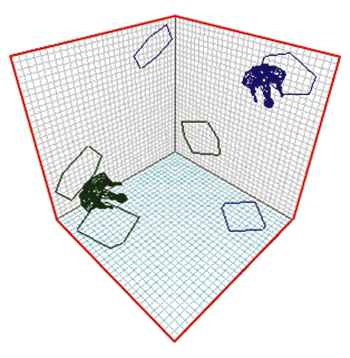FIGURE 1. The volume of the root node.

This is the bounding cube for all the surfaces, and it is assigned to the root node of the octree. Figure 1 represents two spaceships in a volume of space. Note the use of shadow outlines against the background grids to make it easier to see the position of the ships. Figure 1 is the root node of the tree that holds the entire volume.

Next, select a subset of the surfaces based on an object hierarchy construction algorithm (OHC). This algorithm determines which surfaces are selected, in what order they are processed, and how many are allowed in each subset. You can choose from a number of different algorithms, depending on the needs of your game. In this example, I'll use a simple OHC that selects and groups surfaces based on the volume they occupy. The OHC randomly selects any surface from the scene, which I'll call the "seed surface," as it's the first surface from which a single branch of the Octree grows. The algorithm then finds the nearest surface to the seed surface. If both surfaces fit within a volume of space less than a predefined limit, then both surfaces belong to the same set. We call this predefined limit the "limit volume." In this example, we'll use a simple limit volume that is just a bounding box; more complex shapes can be used, depending on the characteristics of the game. The initial limit volume is usually large enough to contain the largest free-standing object in your scene.

You continue adding the nearest surfaces to the set until the total volume of the set reaches or exceeds the limit volume. All of these surfaces are assigned to a child node of the tree. This node also stores the coordinates of the volume occupied by these surfaces. Select another seed surface from the remaining surfaces in the scene and repeat this procedure until all surfaces in the scene belong to a set and are included in the tree. You have now completed the first level of the tree.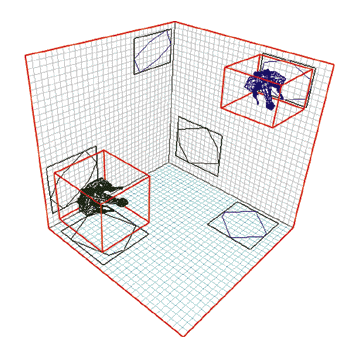FIGURE 2. Limit volumes forming nodes on the tree.

Figure 2 shows the limit volumes around each of the ships. Note that the orientation of the left ship causes it to occupy a larger limit volume than the right ship. This difference is caused by our selection of a bounding-box OHC algorithm; other algorithms can provide tighter fits. Figure 2 is the tree with nodes for each of the ships.

Now we reduce the size of the limit volume, usually by splitting it in half, and recursively repeat the process. Each branch of the octree now points to a single set of surfaces. You repeat the seed-surface selection and subset creation for each of the branches of the octree, which adds a new level to the tree. Figure 3 shows the smaller limit volumes around components of the ships. For clarity, Figure 3 shows only two of the lowest level limit volumes for each ship.

Repeat this process recursively until the limit volume reaches the size of the smallest surface, or until every surface occupies its own branch of the tree. You may have to split some surfaces to accommodate the single surface per branch rule.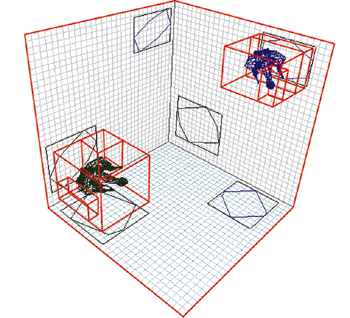FIGURE 3. Further reduction of the limit volumes.

If you allow the algorithm to continue until the limit volume reaches the size of the smallest surface, your scene will display correctly, but it may take too long to create the octree. Using a limit volume that allows more than one surface into each branch improves performance, but sacrifices final quality. In this example, I used a bounding box limit volume, which improves performance of collision detection and object motion, but reduces that of ray tracing.

How you select the seed surfaces will determine the number of child nodes at each level of the tree. Ideally, you want a seed surface to be at the center of a cluster of surfaces in order to reduce the number of nodes in a level. Rubberbanding algorithms that find the center of a cluster are very useful in selecting an optimal seed surface. Seed surfaces that are too isolated from their neighbors generate too many nodes at certain levels, which in turn increases search times when doing collision-detection or ray-tracing calculations.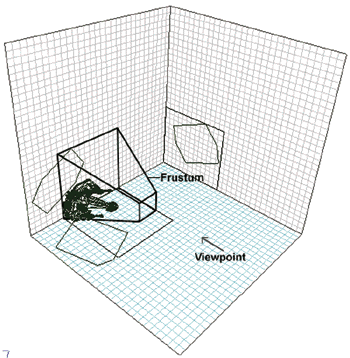FIGURE 4. The camera's viewing frustrum.

Using the Tree

Once the n-tree is complete, you have created a data structure that describes the entire scene in an efficiently increasing level of detail. Now you can use this tree to determine which surfaces are visible from any given camera position. First, calculate the viewing frustum of the camera (Figure 4). Then, determine whether the volume of the viewing frustum intersects with the volume described by each of the branches of the Octree that represent a bounding box (Figure 5). If the two volumes intersect, then recursively follow each branch, checking whether the child nodes intersect the frustum. Move down each node in the tree until you reach the leaf nodes. You now have a path through the n-tree that contains only those surfaces that are included in the frustum (Figure 6). These surfaces can now be fed to a Z-buffer or other rasterizer.

This technique also can be used to obtain line-of-sight calculations.The line-of-sight is treated as a degenerate frustum or is tested for intersection with a volume. Collisions can be calculated by treating the path of an object as a line through the scene and applying the line-of-sight technique.

Handling moving objects with octrees is a little more awkward. If you include moving objects in the algorithms described previously, you will spend a lot of time adjusting the subsets of the tree to include or exclude the moving object from a particular node. Depending on your application, it may be faster if you consider each moving object as a separate and independent leaf node, regardless of the size of the volume it represents. This moving-object leaf node can then be attached to a static node as it moves through the scene.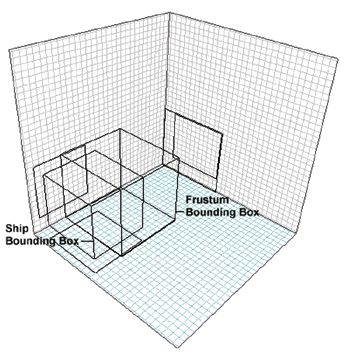FIGURE 5. The intersection of the viewing frustrum with a bounded volume described by a node on the Octree.

Canonical Space Subdivision

The Canonical Space Subdivision form of the OSP algorithm is ideal for applications where most of the space is occupied by objects, and the objects are all approximately the same size (for instance, in maze-type games). The purpose of this algorithm is to manage spatial information about the scene using an octal-tree structure (in which each parent node has eight child nodes) to store its data.

The root node of this tree contains all of the surfaces in the scene. Each branch node contains an octant (one-eighth) of the parent space until you reach a level where each octant is the size of the smallest surface in the scene.

Creating the Tree

To create the initial tree, first obtain the maximum dimensions of the scene that you wish to manage. Adjust the dimensions to form a cube. This is the bounding cube of the entire scene and is assigned to the root node of the tree (Figure 1).

Next, subdivide the bounding cube into eight smaller cubes, known as cubelets. For clarity, Figure 7 shows the cubelets represented by red lines against the background grids. Each one of these cubelets will be held in a child node. If the cubelet contains any surfaces, assign the surfaces to the node. If a surface straddles more than one cubelet, split the surface into subsurfaces. Store the coordinates of the cubelet in the node. Assign an identification number to each cubelet based on the octant it represents (the upper-left-nearest cubelet is assigned number 1, the next cubelet number 2, and so on).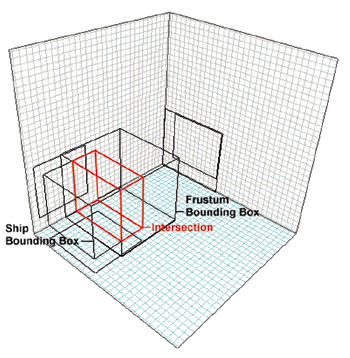FIGURE 6. Viewing path cutting through the tree.

Repeat this procedure (Figure 8) until the size of the cubelet reaches a predefined lower limit (usually the size of the smallest surface), or until each cubelet contains a single surface. The tree is now complete.

Using the Tree

Once your tree is complete, you have a data structure that describes the entire volume of space in a regular, hierarchical form. You can use this data structure to determine which surfaces are intersected by a given line, say the path of a missile or the viewer's line of sight (Figure 9). Obtain the two intersections of the given line with the root cube of the tree, and then select one of these intersections as the start position of the algorithm. Traverse the tree until you find the smallest cubelet that contains the start position. If there are any surfaces in the cubelet, test for intersection of the line with the surfaces in the cubelet.

Using this starting cubelet and the 3D slope of the line, you can obtain the next cubelet that the line passes through using a 3D variation of Bresenham's algorithm. This variation is simply the same 2D line-drawing algorithm extended to 3D and is termed a "3D Digital Differential Analyzer" (3DDDA). Repeat this procedure until you reach the other endpoint of the line.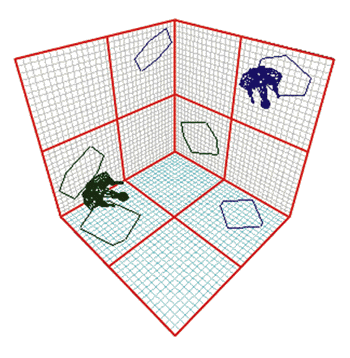FIGURE 7. Dividing the volume into cubelets.

You can extend this technique to determine what surfaces are inside the viewing frustum. First, obtain the eight endpoints that define the limits of the viewing frustum. Then, obtain the slopes of the lines defined by the points. Traverse the tree until you find the smallest cubelets that contain the endpoints of the lines. Select the four cubelets that contain the endpoints of the lines that define the near clipping plane of the viewing frustum. Using the slopes of the frustum lines and the endpoint cubelets, apply the 3DDDA to obtain all the cubelets through which the near clipping plane passes. Use these cubelets, the remaining slopes and endpoints, and the 3DDDA to obtain the remainder of the cubelets in the frustum. Pass all the surfaces contained in the cubelets to the rasterizer.

Handling moving objects is fairly straightforward. If you set the predefined lower limit of the cubelet to an integral factor of the smallest distance an object can travel in the world, keeping track of which cubelet contains the object becomes a simple matter of applying the 3DDDA.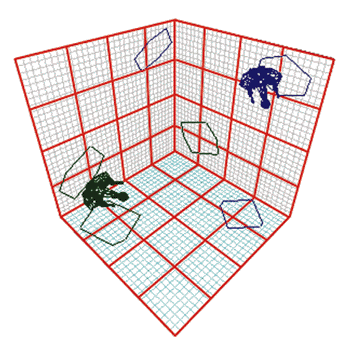FIGURE 8. Further subdivision into cubelets.

Using the octree algorithms as the centerpiece of a game lets you display true 3D objects with a high degree of visual precision and performance. Using these algorithms correctly can give you enough extra horsepower to add a little transparency, or real-time physics, providing the difference between just another game and a beautiful work of art.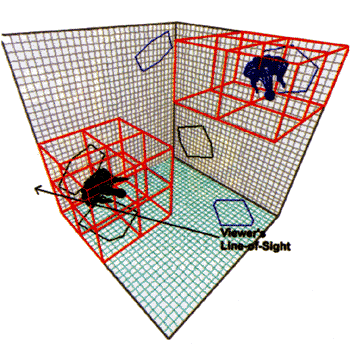FIGURE 9. Determining line of sight in an Octree structure.

Mike Kelleghan builds 3D real-time articulation engines for various game companies in Los Angeles, and is currently recovering from a case of tendonitis caused by too much joysticking on flight simulators. He has, so far, been unsuccessful in convincing the insurance company that it should buy him a more ergonomic joystick.

### Latest Jobs

#### Treyarch

Playa Vista, California
6.20.22
Audio Engineer

#### Digital Extremes

6.20.22
Communications Director

6.20.22
Senior Producer

#### Build a Rocket Boy Games

Edinburgh, Scotland
6.20.22
More Jobs